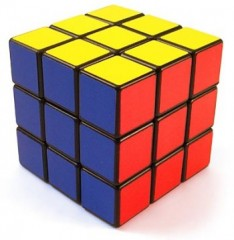Mark Mottian answered Dan Banks' questionI suggest you start here. It is an very informative video which teaches you step by step together with multiple algorithms required for effortlessly solving the Rubix Cube. It takes a lot of memorising but is, however, very satisfying in the end. Go ahead and view part two once … Read more
favorited this question.
Mark Mottian answered August Lavender's question
The following divisibility rules may be important for the nature of such a problem. For divisibility by 2, the units digit of the number should end in either 0; 2; 4; 6 or 8.For divisibility by 3, the sum of the digits of the number should be divisible by 3.For divisibility by 5, the units … Read more
favorited this question.
Mark Mottian answered Burgon Shuler's question
P = (number of favourable outcomes) / (total number of outcomes)Total number of outcomes  = 2 Nickels + 3 Pennies + 3 Quarters + 2  Dimes = 10 CoinsWe are interested in the probability of selecting dime = 2/10 = 1/5